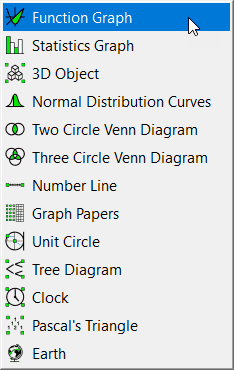﻿ Function Graphs

# Function Graphs

Top  Previous  NextThe graph tool allows you to draw graphs of Cartesian, polar, parametric, implicitly defined and inverse functions.

## FX Draw shares the graphing tool with FX Graph and a full discussion on how to use it is included in Quick Entry Screen

Available Parameters for Function Graphs

\$f1                - definition of function

\$point                - coordinates of point of interest

when annotating a point of intersection

\$f2                - definition of second function in intersection

when annotating an integral

\$integral        - definite integral

\$equation        - definition of integral function

\$area                - area of definite integral

\$upper        - upper bound of integral

\$lower                - lower bound of integral

when annotating a tangent line

\$slope                - gradient of tangent line

\$equation        - definition of derivative function

\$tangentline        - equation of tangent line

\$at                - point at which tangent line touches function

\$intercept        - y intercept of tangent line